Impact Factor 1.895 | CiteScore 2.24
More on impact ›

# Frontiers in Physics## Original Research ARTICLE

Front. Phys., 30 April 2020 | https://doi.org/10.3389/fphy.2020.00105

# Evaluating the Performance of Ultra-Low-Field MRI for in-vivo 3D Current Density Imaging of the Human Head

• 1Physikalisch-Technische Bundesanstalt, Berlin, Germany
• 2Department of Neuroscience and Biomedical Engineering, Aalto University School of Science, Espoo, Finland
• 3Institute of Biomedical Engineering and Informatics, Technische Universität Ilmenau, Ilmenau, Germany

Magnetic fields associated with currents flowing in tissue can be measured non-invasively by means of zero-field-encoded ultra-low-field magnetic resonance imaging (ULF MRI) enabling current-density imaging (CDI) and possibly conductivity mapping of human head tissues. Since currents applied to a human are limited by safety regulations and only a small fraction of the current passes through the relatively highly-resistive skull, a sufficient signal-to-noise ratio (SNR) may be difficult to obtain when using this method. In this work, we study the relationship between the image SNR and the SNR of the field reconstructions from zero-field-encoded data. We evaluate these results for two existing ULF-MRI scanners—one ultra-sensitive single-channel system and one whole-head multi-channel system—by simulating sequences necessary for current-density reconstruction. We also derive realistic current-density and magnetic-field estimates from finite-element-method simulations based on a three-compartment head model. We found that existing ULF-MRI systems reach sufficient SNR to detect intra-cranial current distributions with statistical uncertainty below 10%. However, the results also reveal that image artifacts influence the reconstruction quality. Further, our simulations indicate that current-density reconstruction in the scalp requires a resolution <5 mm and demonstrate that the necessary sensitivity coverage can be accomplished by multi-channel devices.

## 1. Introduction

Imaging of current-density distributions, produced by injecting current in vivo into the human head, has a variety of possible applications. Three-dimensional conductivity distributions or simplified conductivity models may be extracted from such images. These are required for accurate source estimation in electromagnetic neuroimaging [1, 2]. Further, individual conductivity information is necessary for models used to optimize and plan therapeutic treatments, e.g., in transcranial magnetic stimulation (TMS) [3, 4] and transcranial direct-current stimulation (tDCS) . In addition, the current flow during tDCS may be monitored online, providing direct feedback.

Magnetic resonance imaging (MRI) is affected by local magnetic fields, such as the magnetic field BJ(r) associated with a current density J(r) at points r in the imaging volume. In particular, if also the main magnetic field B0 can be switched on and off during the pulse sequence, it is possible to measure full-tensor information of the effects of BJ(r), providing a way to directly estimate J(r) [6, 7]. The field switching can be achieved [8, 9] in ultra-low-field (ULF) MRI, where the main field is not produced by a persistent superconducting magnet as in conventional high-field MRI. Zero-field-encoded current density imaging (CDI) using superconducting quantum interference device (SQUID)-based ULF MRI was first proposed by Vesanen et al. . It has recently been demonstrated in phantom measurements and is most promising regarding in-vivo implementation . Since current impressed in vivo in the human head is limited by safety regulations to the low-mA range [10, 11] and only a small fraction of the current passes the relatively highly-resistive skull [12, 13], a sufficient signal-to-noise ratio (SNR) may be difficult to reach.

The two main factors influencing the SNR in ULF MRI are system noise and the strength of the polarizing field that creates the necessary sample magnetization. Both issues have been addressed in previous setups. However, the ultimate sensitivity combining the lowest noise and the highest polarizing field in a single setup has not been demonstrated. Hömmen et al. used an ultra-sensitive single-channel SQUID system with a noise level of 380 $\mathrm{\text{aT/}}\sqrt{\mathrm{\text{Hz}}}$ for the demonstration of CDI . This noise performance was about 10–20 times better than in commercially available SQUID systems, but the polarizing field of 17 mT was comparatively low. Other groups reported ULF-MRI systems with polarizing fields over 100 mT, using cooled copper-coil setups [14, 15]. Even higher polarizing fields could be reached by means of superconducting polarizing coils as presented by Vesanen et al.  and Lehto .

A quantitative survey of the necessary SNR for zero-field-encoded CDI with a defined uncertainty is still pending. In this work, we investigate the influence of noise on the quality of the BJ and J reconstructions by analytic approximations and by means of Monte-Carlo simulations. Our results enable the estimation of the required image SNR for a given statistical uncertainty in the field reconstructions. They further provide an intuitive method to assess the performance of a specific system for current-density imaging.

In addition, two existing ULF-MRI setups are examined more closely regarding their performance in a CDI application. The first is the single-channel setup of PTB, Berlin, described by Hömmen et al. , which is now equipped with an updated polarization setup specially designed for the shape of the human head. The second setup is a whole-head multi-channel system, a successor of the one described by Vesanen et al. , located at Aalto University, Helsinki. The latest version comprises an optimized superconductive polarizing coil , an ultra-low-noise amplifier for flexible switching of all MRI fields , and newly developed SQUID-sensors specially designed for pulsed-field applications .

Realistic BJ and J distributions were derived from finite-element-method (FEM) simulations using a three-compartment head model. Combined with nominal gradient fields and sensitivity parameters of the described setups, the BJ distributions were put into a Bloch equation solver that emulates complete gradient-echo sequences in the time domain. Our simulation results not only provide a good estimate of the statistical uncertainty in zero-field-encoded CDI with currently available technologies but also reveal other important requirements in terms of sample coverage and image resolution.

## 2. Zero-Field-Encoded CDI

To understand the effects of noise, we recap the sequence and reconstruction method designed by Vesanen et al. . More detailed information on the experimental implementation, including the sequence diagram, can be gleaned from Hömmen et al. .

At first, magnetization is built up by a polarization period. Subsequently, all MRI fields are turned off and the current density J is applied during a defined zero-field time τ. After the zero-field time, the magnetization has been rotated to m1 by the magnetic field during τ as

where m0 is the starting magnetization and γ is the gyromagnetic ratio of the proton. A is the generator of the rotation matrix Φ, which describes the magnetization dynamics due to the quasi-static magnetic field during τ [19, p. 86–89] . Ideally, this field is solely determined by the magnetic field BJ associated with J. In reality, a superposition of a static background field and transient fields due to pulsing (in the following combined in the term BB) are present. Hence, the time evolution of m is affected by AJ + AB, where AJ and AB are associated with BJ and the average BB, respectively.

Following τ, the main field B0, here in the x-direction, is turned on and the magnetization is manipulated by gradient fields to encode spatial information in the phase and frequency of the resulting signal. Ignoring relaxation, the magnetic signal recorded at a sensor during the echo can be written as

where t is the time, C the coupling field of the sensor, and matrices Rf and Rp correspond to rotations in the yz plane during the frequency- and phase-encoding periods. For the following operations, it is convenient to convert the signal equation to a complex representation. Considering only the frequency components close to the Larmor angular frequency γ|B0|, the signal can be written as [20, 21]

where β = Cz + iCy, ${\stackrel{~}{m}}_{1}={m}_{\mathrm{\text{1}},z}+i{m}_{\mathrm{\text{1}},y}$, ωt is the phase angle due to precession during frequency encoding, and θp the angle due to phase encoding. In a realistic setting, β could also include additional effects from an inhomogeneous polarizing field and non-idealities in field pulsing.

After applying the discrete Fourier transform to the frequency- and phase-encoded data and taking the relevant frequency bins, the magnitude and phase of the rotation of m can be estimated at the location of the corresponding voxel. The voxel value corresponding to the MR signal generated close to rn is given by

where SRF(rrn) is the spatial response function of the nth voxel . When the SRF is close to a delta function δ(rrn), the integral can be approximated with the function value at rn, otherwise the SRF will result in leakage artifacts from the neighboring areas.

The voxel values vn contain information about the zero-field-encoded magnetic field in both their magnitude and phase. In reality, there are other factors, such as non-idealities in the gradient ramps and unknown relaxation profiles, that affect the voxel values as well. Therefore, the relative changes in vn associated with the current density are recovered by normalization with a reference un [6, 9]. Repeating the sequence for three orthogonal starting magnetizations m0, x = |m0|ex, m0, y = |m0|ey and m0, z = |m0|ez (shown in Figure 1), the last two rows of Φn can be measured. For example, the y and z elements of the first column are given by:

where νn, x denotes the voxel value of a zero-field-encoded image with starting magnetization in the x direction. Rotation matrices are orthogonal by definition. Therefore, the first row of Φn can be derived by the cross product of the second with the third row. Naturally, the elements in Φn are contaminated by noise. A practical approach to increase the accuracy is to apply an orthogonalization. For this purpose Vesanen et al.  suggest Löwdin's transformation, which yields the closest orthogonalization in the least-squares sense [23, 24]. It is clear that a unique rotation matrix Φn is created for each voxel n. The following analysis in this section and in section 3 concentrates on a voxel-wise reconstruction of BJ and J, where the index n is left out for simplicity.

FIGURE 1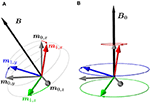Figure 1. (A) Rotation of three orthogonal starting magnetizations m0, x = |m0|ex, m0, y = |m0|ey, and m0, z = |m0|ez about the direction of B = BB + BJ during the zero-field time. (B) After the zero-field time, the main field B0 = |B0|ex (|B0|≫|B|) is turned on and the vectors start rotating about ex.

Using Φ, all components of the magnetic field B = BB + BJ can be derived from a non-linear inversion of the matrix exponential:

where ϕ = arccos[(tr(Φ) − 1)/2] represents the rotation angle of Φ , and $\stackrel{^}{B}$ is the reconstruction of B. From here on, reconstructed quantities are denoted using the hat symbol.

Finally, BJ can be estimated by subtracting another reconstruction from $\stackrel{^}{B}$. This could be a full 3D image of BB only, or of BB + BJ with the impressed current having the opposite polarity. The latter reduces the statistical uncertainty by $1/\sqrt{2}$ and is from here on called bipolar reconstruction:

From Equation (7), the full tensor of the local field ${\stackrel{^}{B}}_{J}$ is derived, enabling the estimation of $\stackrel{^}{J}$ by Ampère's Law:

where μ0 is the permeability of free space.

## 3. Noise in Zero-Field CDI

### 3.1. The Connection Between Noise in Φ and Image SNR

In this section, we analyze how the uncertainty in the reconstruction of zero-field-encoded data relates to the image SNR. From Equation (5), we know that the values in Φ are normalized by a complex reference u = |u|e, where |u| is related to the magnitude of the magnetization after τ and δ to the phase accumulation due to effects that do not arise from BJ + BB.

Hömmen et al.  describe that |u| cannot be measured directly due to the always present background field. However, the reference can be constructed from the real or imaginary parts of the three measurements of v by

which effectively normalizes the rows of Φ to exactly unit norm. The reference phase δ, on the other hand, has to be acquired in a separate measurement. See Hömmen et al.  for more detail.

The complex reference value can be modeled as u = E[u] + ϵ, where E denotes the expected value and $ϵ~{N}\left(0,{\sigma }^{2}\right)$ is symmetric complex Gaussian noise that can be extracted from a noise-only image e, or from a noise-only region in any of the images v. Using this reference, we define the image SNR as

where SD is the standard deviation.

The phase correction with the noisy reference phase δ causes the real part to leak to the imaginary part and vice versa, increasing the noise in the matrix elements. Dividing by the magnitude of the complex reference u = |u|e yields unit norm in the rows of Φ decreasing the noise. This is derived in the Appendix, which also shows that the noise SD in the elements of Φ can be approximated as

where the scaling $1\le {g}_{ij}\left(\Phi \right)\le \sqrt{2}$ depends on the associated measurement. This approximation is valid when u ≈ E[u], i.e., SNR ≫ 1. Equation (11) already gives an impression of the noise SD in Φ as a function of the image SNR. The rotation-dependent scaling gij(Φ) and correlations between the elements are given in the Appendix.

The most important factors determining the SNR are the polarizing field, the coupling to the sensors, and the relaxation of the magnetization, all of which affect the voxel magnitude. The noise in the voxel values is governed by the system noise determined by the magnetic sensor as well as other instrumentational and environmental noise sources.

### 3.2. Noise Analysis of B-Field Reconstruction: Linear Approximation

To estimate the noise in the reconstruction of B, we first discuss an idealized case, where all three rows of Φ can be measured and no reference image u is needed. In this case, the noise in the elements of Φ becomes independent and identically distributed with standard deviation of .

We start by using a first-order small-angle approximation of the rotation matrix

where I is the identity matrix. The magnetic field components can be solved directly and, as each component is measured twice, they can be averaged so that the noise SD in the angular quantity becomes . Here, d is any of the components x, y or z, and the noise SD of a magnetic field component can be derived to .

In reality, the elements of Φ are estimated with the help of a reference image, which modifies the noise in the elements as derived in the Appendix. Additionally, only two rows of the rotation matrix Φ can be obtained from the measurements as explained in section 2. Therefore, one row (in our case the first row) has to be derived from the cross product of the adjacent rows, where the cross product contains information about the components of B orthogonal to the direction of B0. These components are no longer subject to independent random noise; consequently, the noise is not reduced by the averaging effect in the linear reconstruction.

So far, the noise analysis was discussed for the reconstruction of the effective B-field. As mentioned before, in practice, the measurement of BJ is contaminated by a background field BB, which must be eliminated by subtracting a second reconstruction. The noise in the two reconstructions is independent, which is why in the case of bipolar reconstruction the noise in the field estimate is reduced by a factor of $\sqrt{2}$ (see Equation 7). Additionally, as the reference phase δ is the same for the two data sets, the additional noise due to referencing will cancel in the field subtraction.

In the first-order approximation, we finally obtain for bipolar reconstruction

and

because Bx is measured twice.

### 3.3. Noise Analysis of B-Field Reconstruction: Monte-Carlo Simulations

From the first-order small-angle approximation we can gain intuitive understanding of the statistical uncertainty in the reconstruction of BJ. However, in reality, the rotation angle ϕ can obtain values up to π and the linear approximation breaks down.

In order to estimate the influence of noise on the non-linear reconstruction, we carried out a series of Monte-Carlo simulations. Therefore, we generated the last two rows of rotation matrices Φ for 100 different rotation angles ϕ = ±γτ|B| taken uniformly between −π < ϕ < π, where the negative angles correspond to −B. As before, B = BB + BJ, where BJ was set to zero and ϕ was varied by adjusting BB. The matrices Φ were generated using the general formula of Rodriguez, as explained in [19, p. 86–89]:

Here, K = γτA/ϕ is a unitary cross-product matrix associated with the rotation axis. Independent and Gaussian-distributed random noise was generated and superimposed with each element of Φ, according to Equation (11). Subsequently, the first row was derived by the cross product of the other two. The procedure was repeated 100,000 times to obtain statistics for the reconstruction quality.

Figure 2 illustrates the standard deviation after three intermediate steps of the reconstruction, showcasing their influences on the result. The data are normalized to the input noise corresponding to Equation (11) without gij(Φ).

FIGURE 2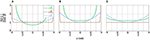Figure 2. Single-voxel Monte-Carlo simulations to estimate the influence of noise on three different steps of the non-linear reconstruction as a function of the rotation angle ϕ. The shown data are based on simulated noisy rotation matrices, where the first row was derived by the cross product of the other two. Displayed are normalized standard deviations of each component of $\stackrel{^}{B}$, which is the reconstruction of y-directional field B = |BB|ey. |BB| was adjusted to generate the rotation angles ϕ with the negative angles corresponding to the field direction −ey. The main field B0 was x-directional. The figures show the standard deviations of reconstructions without pre-referencing (A), with pre-referencing (B), and with subsequent orthogonalization using Löwdin's transformation (C).

Figure 2A illustrates a case where no referencing with u was applied. Each element of Φ thus contained the same amount of Gaussian distributed noise. Although this may not be the case in an experimental implementation, one sees that ${\stackrel{^}{B}}_{x}$ contains $1/\sqrt{2}$ the noise of the other components for small angles of ϕ, as predicted by the first-order approximation. However, with a rising field strength, i.e., larger rotation angle ϕ, the noise in this component increases non-linearly and more strongly compared to the components orthogonal to B0.

The simulations underlying Figure 2B include the necessary pre-referencing. For very small angles, the extra phase noise due to the noisy reference phase δ affects the noise SD only in ${\stackrel{^}{B}}_{x}$. Toward larger angles, this effect is visible in ${\stackrel{^}{B}}_{z}$. The y-component of $\stackrel{^}{B}$ is not affected, which is in accordance with the analysis presented in the Appendix.

Figure 2C shows the results after subsequent orthogonalization using the Löwdin transformation. We observe a strong effect toward large angles ϕ, especially in the x-component, which is parallel to B0.

Figure 3 illustrates the standard deviations of the results of a simulated bipolar reconstruction. In comparison to Figure 2, these data sets are arithmetic means of two similar fields (independent noise, identical reference), respectively Equation (7) with BJ = 0. Overall, the noise levels decrease by a factor of $\sqrt{2}$, in comparison to the reconstructions of the effective field B in Figure 2. Further, the additional noise due to the reference phase δ, visible in Figures 2B,C, was subtracted entirely. Except for very large angles (ϕ > 7π/8), the noise SD in each component is lower than . Figure 3 also shows a measure to assess the expected deviation from the mean of ${\stackrel{^}{B}}_{J}$ (purple line), which can be derived to be the square root of the trace of the covariance matrix:

FIGURE 3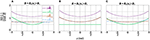Figure 3. Single-voxel Monte-Carlo simulations to estimate the standard deviation of each component of ${\stackrel{^}{B}}_{J}$ after bipolar reconstruction (Equation 7), in dependence of the rotation angle ϕ. In addition, $\sqrt{tr\left[cov\left({\stackrel{^}{B}}_{J}\right)\right]}$ (Equation 16) is presented in purple, dash/dotted lines. B is the effective field BB + BJ, where BJ was set to zero and BB was adjusted to generate defined rotation angles ϕ with negative angles corresponding to −B. The figures represent reconstructions, where BB was y-directional (A), x-directional (B), and diagonally oriented in ${e}_{\mathrm{\text{d}}}=\left[1,1,1\right]/\sqrt{3}$ (C). The main field B0 was x-directional in all cases.

### 3.4. Noise Analysis of Current-Density Reconstruction

From the noise in the reconstruction of BJ, we can also calculate the noise in the current density reconstruction using Equation (8). For that, we make some simplifications. We assume a constant current density in a homogeneous and isotropic medium. Further, we assume a homogeneous background field that is much larger than BJ. A simple method for the spatial derivation is to take into account only the two nearest neighbors at zl and z + l

where z is the coordinate of the voxel in the z-direction and l is the voxel sidelength. Assuming equal SNR at z + l and zl, the noise SD of the gradient is approximately ${\sigma }_{G\left(z\right)}={\sigma }_{{\stackrel{^}{B}}_{J}\left(z\right)}/\left(l\sqrt{2}\right)$. Applying the curl

and neglecting the small possible differences in ${\sigma }_{{\stackrel{^}{B}}_{J,z}}$ and ${\sigma }_{{\stackrel{^}{B}}_{J,y}}$, the noise SD of Ĵx can be approximated as ${\sigma }_{{Ĵ}_{x}}={\sigma }_{{\stackrel{^}{B}}_{J,z}}/\left(l{\mu }_{0}\right)$.

### 3.5. Field Reconstruction Quality in Terms of Image SNR

Using the definition of image SNR in Equation (10) and the results of the Monte-Carlo simulations, the signal-to-noise ratio of the BJ reconstruction () can be estimated by

where $SD\left[{\stackrel{^}{B}}_{J}\right]$ is the measure for noise in the vector ${\stackrel{^}{B}}_{J}$ defined in Equation (16). Further, the scaling factor c depends on the strength and the orientation of BB and can be read directly from the purple, dash/dotted lines in Figure 3. As c is highest for x-directional background fields, a polynomial, normalized to 1/π, was fitted to the data presented in Figure 3B, to approximate c as a function of ϕ:

Note that the results presented in Figures 3A,C only deviate slightly from Equation (20).

According to the figure, without any information on the background field, a representative value for the scaling factor would be c = 1.3. This is close to the worst-case scenario as higher rotation angles may cause phase wrapping.

To provide a numerical example, let us assume that |BJ| = 10nT, a homogeneous x-directional background field of 60 nT, and a zero-field time of τ = 100 ms, taking into account the T2-relaxation time of gray matter in the μT regime of approximately 100 ms. Substituting the rotation angle ϕ = γτ|B| in Equation (20), c is approximated to be 1.2. According to Equation (19), for a required , the voxel SNR needs to be over 32.

The estimation of J using Ampère's law requires the determination of local field gradients, where the noise in the reconstruction is inversely proportional to the voxel side length l. This effect should not be underestimated; as the signal strength already scales to the voxel volume l3, the SNR of $\stackrel{^}{J}$ scales to the fourth power of the voxel sidelength. The quality of the J-reconstruction can be determined from the SNR of ${\stackrel{^}{B}}_{J}$, by including the scaling factor 0 in Equation (19):

The approximation in Equation (21) is valid when the voxels involved in the gradient estimation are subject to equal SNR. Especially at tissue boundaries, this can cause erroneous assessments due to different relaxation times.

Again, to provide an example, we assume a current density distribution of 0.4 A/m2, a value in accordance with the literature for a stimulation of approximately 4 mA . Similar to the example above, c ≈ 1.2 is assumed. If we want to derive $\stackrel{^}{J}$ with SNR[$\stackrel{^}{J}$] > 10 and a voxel-sidelength of 5 mm, a required image SNR of 130 is estimated.

## 4. Simulated Performance of ULF-MRI Systems

### 4.1. MRI Simulation Setup

The main factors that determine the SNR profiles of ULF-MR images are the sensor arrangement, system noise, and the polarizing field profile. To evaluate the sensitivity of the ${\stackrel{^}{B}}_{J}$ and $\stackrel{^}{J}$ field reconstruction in a realistic situation, we set up a simulation toolbox incorporating realistic polarizing fields and sensor geometries, as well as time-domain magnetization evolution based on analytical solutions of Bloch's equation. Assuming ideal gradient fields and instantaneous field switching, gradient-echo sequences can be simulated for arbitrary imaging objects. Both the polarizing field profile and the coupling of the magnetization to the sensor (Equation 2) were calculated by analytically integrating the Biot–Savart formula over line segments [20, 25].

Two sets of simulations were set up to correspond to the single-channel system with a wire-wound 2nd-order axial gradiometer and a resistive polarizing coil as present at PTB, Berlin, and the multi-channel whole-head system with 102 planar thin-film magnetometers and a compact superconducting polarizing coil built at Aalto University (see Figure 4). Based on measured values, the sensor noise in the single-channel system was set to 350 $\mathrm{\text{aT/}}\sqrt{\mathrm{\text{Hz}}}$ and in the multi-channel system to 2 $\mathrm{\text{fT/}}\sqrt{\mathrm{\text{Hz}}}$. A polarizing current of 50 A was chosen for both setups corresponding to field maximum of 90 mT and mean of 65 mT in the brain compartment for the single-channel system. For the multi-channel system, the field maximum was 115 mT and the mean 70 mT in the brain compartment.

FIGURE 4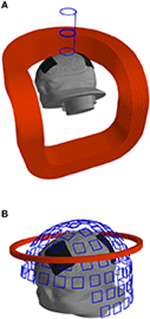Figure 4. Geometries of the single-channel MRI setup at PTB (A) and the multi-channel MRI setup at Aalto (B). The illustrations include the polarizing coil (red), the receiver coils of the sensors (blue), the head model (gray), and the stimulation electrodes (black).

For the evaluation of the simulations, a comparison with actual measurements using the PTB setup was executed. Therefore, a spherical single-compartment phantom (80 mm diameter), filled with an aqueous solution of CuSO4+H2O to tune the T2-relaxation time to approximately 100 ms, was placed with a gap of 10 mm below the dewar (nominal warm-cold distance 13 mm). The current in the polarizing coil was set to 20 A, resulting in an inhomogeneous polarizing field of approximately 25 mT. Gradients were set to give a voxel size of (4.8 × 4.8 × 4.8) mm3 and a field of view (FOV) of 115 mm in the phase-encoded directions y and z. The resulting time signals of the gradient echos were processed to form an array of k-space data. To reduce Gibbs ringing, both the frequency- and the phase-encoding dimensions were tapered with a Tukey window (shape parameter = 0.5) and the three-dimensional FFT was applied to reconstruct the images. For the simulations, the sphere was approximated by a regular 1-mm spaced grid.

Figure 5 illustrates the setup, accompanied by magnitude images of measurement and simulation. The results reveal a difference in the amplitude of measured and simulated MRI of approximately 25%, probably subject to multiple origins. A shielding coil reduces the polarizing field of the actual setup, which was not accounted for in the simulations. Also, winding errors due to the relatively complex geometry of the polarizing coil reduce the current–field ratio. In addition, the true warm–cold distance of the dewar could vary depending on the helium level and the phantom mount also might have inaccuracy in the mm range. Taking all these uncertainties into account, the simulated MRI sequence resembles the realistic conditions found in actual measurements.

FIGURE 5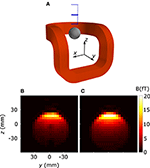Figure 5. Comparison of measured and simulated MRI images. (A) shows the utilized setup, including the polarizing coil (red), the spherical phantom (gray), and the receiver coil of the sensor (blue). Central slices of reconstructed images, not corrected for the sensitivity profile, are presented for measurement (B) and simulation (C). Please note that the actual phantom contains a mount for dipolar current electrodes, which is recognizable in the central lower half of the reconstructed measurement, but was not accounted for in the simulations.

### 4.2. MRI Simulations With Head Model

In the next step, the simulation setup was used to generate full CDI sequences with the single-channel system, as well as with the multi-channel system, using the BJ distribution derived from finite-element-method (FEM) simulations of a realistic head model. This model is based on CT scans of a human head  and contains three compartments as shown in Figure 6A. The conductivity in the outermost scalp compartment was set to 0.22 S/m, in the skull compartment to 0.01 S/m, and in the innermost brain compartment to 0.33 S/m. The two stimulation electrodes were positioned roughly 10 cm apart, one on the forehead and the other one on the side of the head. The electrode dimensions were (50 × 70)mm2 and their conductivity was set to 1.4 S/m.

FIGURE 6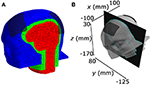Figure 6. (A) Tetrahedral FEM mesh consisting of intra-cranial volume (red), skull (green), and scalp (blue) compartments. The electrodes are illustrated in transparent gray. (B) Model positioning in the MRI coordinate system. The plane between the electrodes corresponds to the slice in Figures 8, 9.

The FEM simulations to obtain the current density J and the resulting magnetic field BJ were conducted in the Comsol Multiphysics software based on the generalized minimal residual method (GMRES). Current flow was realized by setting zero potential on the outer surface of the cathode and applying a total current of 4.5 mA to the outer surface of the anode. For the calculation of BJ, a spherical air compartment (2 m in diameter) was added to the model, ensuring a negligible effect of the magnetic isolation boundary condition.

For the MRI simulations, the head model was positioned in the FOV of the two described scanner arrangements, similar to how the positioning of a head would be in an actual measurement setup (compare with Figure 4). The scalp–sensor distance was 16 mm for the single-channel setup and 20–35 mm for the multi-channel setup, taking into account the individual warm–cold distances of the two systems plus 3 mm to compensate for the amplitude differences found in the comparison with actual measurements, as described in section 4.1. The magnetization was discretized to tetrahedral elements derived from the geometry of the Comsol model. The time evolution of the magnetic moment was simulated for the center of each element. The T2-relaxation time for the brain compartment was set to 106 ms and for the scalp compartment to 120 ms . For simplicity, as the spin density in the skull is insignificant compared to soft tissue, this compartment was assumed to have no magnetization at all. The average tetrahedron sidelengths were approximately 3.5 mm in the brain and 2.5 mm in the scalp. Gradients were set to give a voxel size of (5 × 5 × 5) mm3 and a field of view (FOV) of 220 mm in the phase-encoded directions. Figure 6B presents the head model in the coordinate system defined by the MRI gradients. As performed for the spherical phantom, both the frequency- and phase-encoding dimensions were tapered with a Tukey window (shape parameter = 0.5) before computing the three-dimensional FFT. For the multi-channel system, images of each sensor were combined voxel-wise using the coupling field information as described in Zevenhoven et al. .

### 4.3. Simulation Results

Patterns of the simulated current density and the associated magnetic field, as derived from the FEM simulations, are shown in Figure 7. Due to the low conductivity of the skull, the highest current density can be found in the scalp compartment. In the vicinity of the electrode boundary, |J| was up to 15 A/m2. The maximal current density in the brain compartment below the electrodes was about 0.5 A/m2. In relation to that, the magnetic field appeared smoother, yielding maximal field strengths of 20 nT in the scalp and 12 nT in the brain compartment. The maximum of the field magnitude in the brain compartment is located in between the electrodes, just beneath skull layer. In contrast, the maximal current density in the brain is located beneath the electrodes.

FIGURE 7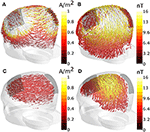Figure 7. The FEM simulation results for current density J are visualized in the scalp (A) and in the brain compartment (C). The simulated magnetic field BJ, due to all current flowing in the head, is plotted in the scalp (B) and in the brain (D). The arrow lengths are scaled logarithmically because of the vast magnitude differences especially in the current density. Each subfigure shows only the top 30 (magnitude) percentile of the field in the respective compartment.

Figure 8 shows a comparison between the field reconstructions $|{\stackrel{^}{B}}_{J}|$ and |$\stackrel{^}{J}$| of the simulated zero-field sequence and ground-truth FEM solutions of |BJ| and |J|. Both data sets are presented without noise. The plane corresponding to the slice is defined in Figure 6B. The reconstructed magnetic field $|{\stackrel{^}{B}}_{J}|$ resembles closely the corresponding FEM solution, which was used as an input to the MR simulations. Notable differences are found inside the skull, which is expected due to the lack of magnetization, as well as on the top parts of the scalp at the field maximum. The difference image reveals ringing artifacts in the intra-cranial volume, leading to error fields up to approximately 1 nT.

FIGURE 8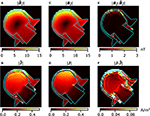Figure 8. Comparison of the simulated noiseless CDI reconstructions and the FEM solutions. The corresponding coordinates are given in Figure 6B. (A) shows the reconstructed magnetic field and (B) the reconstructed current density. (C,D) show the respective FEM solutions, and (E,F) display the absolute differences between reconstructions and the FEM solutions. The FEM fields are linearly interpolated from the FEM nodal values to the (5 × 5 × 5) mm3 voxel grid. The reconstructions are masked to zero outside the head model. Note that the color axes of the right-most figures differ from the others by a factor of 5.

The difference between the reconstructed current density |$\stackrel{^}{J}$| and the corresponding FEM solution of |J| is more prominent. Although no noise was added to the simulated data, errors in the finite-difference approximations and artifacts in $\stackrel{^}{B}$ add up, so that the field estimate near the skull is highly distorted. The intra-cranial fields show greater resemblance, although a notable ringing-artifact from the skull can be seen in |$\stackrel{^}{J}$|.

Figure 9 displays the performance of the two ULF-MRI setups with the simulated imaging sequence described in section 4.1. Figures 9A,B show field reconstruction magnitude $|{\stackrel{^}{B}}_{J}|$ for a CDI sequence with 50 A polarizing current. The time-domain echo signals were superimposed with Gaussian noise of 2 $\mathrm{\text{fT/}}\sqrt{\mathrm{\text{Hz}}}$ and 0.35 $\mathrm{\text{fT/}}\sqrt{\mathrm{\text{Hz}}}$ for the multi-channel system and the single-channel system, respectively. The reconstruction quality is highly dependent on the SNR of the underlying ULF-MR images, which is shown in Figures 9C,D. With the ultra-sensitive single-channel setup, one achieves sensitivity in depth to the intra-cranial volume whereas the multi-channel setup gives a broader sensitivity pattern on the scalp and directly under the skull. Figures 9E,F illustrate estimates of the SNR maps of ${\stackrel{^}{B}}_{J}$, corresponding to the images in Figures 9A,B. The maps are derived from the noiseless ${\stackrel{^}{B}}_{J}$ and the SNR maps using Equation (19) with c = 1.3.

FIGURE 9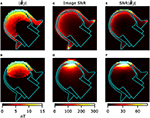Figure 9. Comparison of system performances of the Aalto multi-channel (A,C,E) and the PTB single-channel (B,D,F) ULF-MRI setups. (A,B) show $|{\stackrel{^}{B}}_{J}|$ reconstructions of CDI simulations thresholded above SNR = 20 and inside the head model. (C,D) show the SNR maps of the simulated magnitude images and (E,F) contain estimates of SNRs of $|{\stackrel{^}{B}}_{J}|$ in both systems.

## 5. Discussion

Hömmen et al.  concluded that an increase in image SNR of their setup is necessary for a successful in-vivo implementation of current-density imaging. However, based on measurements using simple phantoms, no exact numbers for the requirements in terms of SNR could be presented.

This work provides a profound understanding of the influence of noise on the reconstruction of the magnetic field BJ and the current density J. The linearization of the field reconstruction gives an approximate relationship between the image SNR and the statistical uncertainty in the field estimates. Further, Monte-Carlo simulations were used to derive the statistical uncertainty in the presence of large background fields where the non-linearities take effect. The presented link between image SNR and noise in the reconstruction allows the determination of the necessary SNR for the reconstructions ${\stackrel{^}{B}}_{J}$ and $\stackrel{^}{J}$ within a predefined uncertainty. It also enables the assessment of the performance of specific ULF-MRI systems for zero-field-encoded CDI directly from acquired or simulated image data.

In order to retain constant image SNR in the Monte-Carlo simulations, we adjusted |BB| to vary ϕ = γτ|BB|. We set the zero-field-encoding time to τ = T2, which yields maximum SNR[BJ] according to Vesanen et al. . However, the non-linear dependence of SNR[BJ] on ϕ suggests that there is an optimum set of parameters for each specific case. In reality, the effective background field will be roughly constant over the measurement periods and τ should be adjusted to obtain maximum SNR[BJ]. If the relaxation times are known, Equations (19) and (20) can be utilized to create a cost function that provides parameters for maximum reconstruction quality. It should be mentioned that the optima for τ are flat and close to T2 for small background fields. An adjustment of τ seems worthwhile in the case of very large background fields, where up to 12% can be gained in compared to τ = T2. Furthermore, it should be kept in mind that ϕ < π should be fulfilled to prevent ambiguity in the field reconstruction.

To analyze their performance and suitability for in-vivo CDI, our two ULF-MRI systems were examined in realistic image simulations. One was the system of Hömmen et al., including an optimized polarizing setup, and the second was a whole-head multi-channel system built at Aalto University. Key features that determine the SNR, such as the polarizing-field pattern, the coupling profile to the sensor, and noise, were accurately modeled. The estimates of BJ and J were derived from FEM simulations using a three-compartment head model. The peak current densities in intra-cranial tissue are similar to literature values, when scaled to the applied current of 4.5 mA [12, 13]. However, the three-compartment model neglects the fact that current is partly shunted by cerebrospinal fluid (CSF), which has a higher conductivity compared to gray- and white-matter tissue [13, 28].

The BJ-field distribution served as an input for MRI simulations, emulating the entire sequence. Taking into account the insights from the Monte-Carlo simulations and the calculated SNR of the single-channel setup, the required improvement in SNR compared to Hömmen et al.  can now be specified. The simulations verify that the optimized polarization profile is sufficient. The peak SNR of the multi-channel setup is lower compared to the single-channel setup due to a higher sensor noise and different field coupling. A broader sample coverage, on the other hand, is provided by the multi-channel setup. The comparison between the two systems revealed that both high sensitivity and large sample coverage are required for current-density imaging usable for conductivity estimation.

It should be mentioned that both systems were evaluated with 50 A of polarizing current, which represents a close to maximum level for the room-temperature coil used with the single-channel device, whereas the superconducting polarizing coil used with the multi-channel device might be able to carry 2–4 times more current. Such an increase in the polarizing current benefits the image SNR and the SNR of the field estimates by the same factor. However, approaching such high fields will cause flux trapping in the sensor [18, 29, 30] and the superconducting filaments of the coil [17, 31], which has to be dealt with. Also larger currents required for the compensation of the field transient  can cause excessive heating in the compensation coils, requiring more sophisticated techniques .

In addition, it should be mentioned that most tDCS devices do not support the application of 4.5-mA current. Nevertheless, some stimulators, such as DC-STIMULATOR PLUS (neuroConn, Germany), support 4.5-mA currents, provided that the electrode–skin resistance is low. The presented estimates for J and BJ, as well as SNR[BJ], scale linearly with the current strength. If the current was reduced to, e.g., 2 mA, the SNR of the single-channel system would still be sufficient to reconstruct BJ in the intra-cranial compartment. However, the reconstruction volume would be reduced. In case of the multi-channel system, the volume of reliable reconstruction would be limited mostly to the scalp compartment. With higher polarizing field, the reconstruction volume would, of course, be recovered again.

Besides noise, spatial leakage from the FFT has a significant influence on the quality of the reconstruction. Appropriate windowing of the k-space data manipulates the spatial response function of the voxels, effectively reducing the far-reaching leakage at the cost of a smoothed resolution. However, with the applied imaging and reconstruction procedures, leakage artifacts could not be entirely eliminated, yielding noticeable reconstruction errors, especially visible in the $\stackrel{^}{J}$-distribution. Besides spatial filtering, an effective method to reduce ringing artifacts in MRI is to apply more k-steps. However, this might not be applicable to in-vivo CDI as it would increase the measurement time significantly. Additionally, post-processing methods, for example “total variation constrained data extrapolation” , might reduce the artifacts without decreasing the image resolution.

The J reconstructions of both systems show limitations in thin tissue structures like the scalp. This is most probably due to the chosen resolution of (5 × 5 × 5)mm3, which does not allow sufficient gradient calculations in these areas. Reducing the voxel size to 1–2-mm would increase the quality of the $\stackrel{^}{J}$-distribution, but again at the cost of longer overall measurement time and lower SNR. Generally, the simulations show that the BJ reconstruction is more reliable than the J reconstruction, as artifacts strongly affect the gradient estimation.

Shall the reconstructions be used to fit individual conductivity values, superior results are expected when the ${\stackrel{^}{B}}_{J}$-field is used as the measurement data. However, magnetic fields arising from the current leads should be either modeled or eliminated from the data. One way to exclude these fields would be to consider only closed path integrals of ${\stackrel{^}{B}}_{J}$ and to apply the integral form of Ampère's law. It remains to be answered whether this enables to derive bulk conductivity values only, rather than spatially resolved conductivity mapping. Methods for this have not been presented so far and should be subject to further research.

## 6. Conclusion

We introduced methods to gain quantitative information about the effect of stochastic uncertainty on the non-linear reconstruction in zero-field-encoded current-density imaging (CDI). The work provides means to determine the ability of specific ultra-low-field MRI setups to reach acceptable signal-to-noise ratios in field reconstructions based on image SNR and to assess necessary improvements in, e.g., noise performance or polarizing field strength. By simulations, we evaluated the reconstruction quality of two existing setups under realistic conditions. We showed that current technology in ULF MRI is suitable for in-vivo CDI in terms of SNR. In addition, we encountered reconstruction errors due to a limited resolution and image artifacts requiring further research and development of more accurate reconstruction techniques.

## Data Availability Statement

The datasets generated for this study are available on request to the corresponding author.

## Author Contributions

PH, AM, and RK contributed to the conception of the study. PH and AM performed the simulations, analyzed the results, wrote the first draft of the manuscript, and revised the manuscript based on the annotations of the co-authors. PH performed comparative measurements. AM contributed to the theory part in the Appendix. AH, RM, and JH developed the head model and performed FEM simulations. All the authors contributed to manuscript revision and read and approved the submitted version. The study was supervised by JH, KZ, RI, and RK.

## Funding

This project has received funding from the European Union's Horizon 2020 research and innovation programme under grant agreement no. 686865. It was partly supported by Vilho, Yrjö and Kalle Väisälä Foundation, by Project 2017 VF 0035 of the Free State of Thuringia, and by DFG Ha 2899/26-1.

## Conflict of Interest

The authors declare that the research was conducted in the absence of any commercial or financial relationships that could be construed as a potential conflict of interest.

## Acknowledgments

The authors thank Jan-Hendrik Storm for fruitful discussions on the FEM simulations.

## Supplementary Material

The Supplementary Material for this article can be found online at: https://www.frontiersin.org/articles/10.3389/fphy.2020.00105/full#supplementary-material

## References

1. Hämäläinen M, Hari R, Ilmoniemi RJ, Knuutila J, Lounasmaa OV. Magnetoencephalography—theory, instrumentation, and applications to noninvasive studies of the working human brain. Rev Modern Phys. (1993) 65:413–97. doi: 10.1103/RevModPhys.65.413

2. Vallaghé S, Clerc M. A global sensitivity analysis of three- and four-layer EEG conductivity models. IEEE Trans Biomed Eng. (2008) 56:988–95. doi: 10.1109/TBME.2008.2009315

3. Opitz A, Windhoff M, Heidemann RM, Turner R, Thielscher A. How the brain tissue shapes the electric field induced by transcranial magnetic stimulation. NeuroImage. (2011) 58:849–59. doi: 10.1016/j.neuroimage.2011.06.069

4. Nummenmaa A, Stenroos M, Ilmoniemi RJ, Okada YC, Hämäläinen MS, Raij T. Comparison of spherical and realistically shaped boundary element head models for transcranial magnetic stimulation navigation. Clin Neurophysiol. (2013) 124:1995–2007. doi: 10.1016/j.clinph.2013.04.019

5. Miranda PC, Callejón-Leblic MA, Salvador R, Ruffini G. Realistic modeling of transcranial current stimulation: the electric field in the brain. Curr Opin Biomed Eng. (2018) 8:20–7. doi: 10.1016/j.cobme.2018.09.002

6. Vesanen PT, Nieminen JO, Zevenhoven KCJ, Hsu YC, Ilmoniemi RJ. Current-density imaging using ultra-low-field MRI with zero-field encoding. Magn Reson Imaging. (2014) 32:766–70. doi: 10.1016/j.mri.2014.01.012

7. Nieminen JO, Zevenhoven KCJ, Vesanen PT, Hsu YC, Ilmoniemi RJ. Current-density imaging using ultra-low-field MRI with adiabatic pulses. Magn Reson Imaging. (2014) 32:54–9. doi: 10.1016/j.mri.2013.07.012

8. Zevenhoven KCJ, Alanko S. Ultra-low-noise amplifier for ultra-low-field MRI main field and gradients. J. Phys.: Conf. Ser. (2014) 507:042050. doi: 10.1088/1742-6596/507/4/042050

9. Hömmen P, Storm JH, Höfner N, Körber R. Demonstration of full tensor current density imaging using ultra-low field MRI. Magn Reson Imaging. (2019) 60:137–44. doi: 10.1016/j.mri.2019.03.010

10. Bikson M, Grossman P, Thomas C, Zannou AL, Jiang J, Adnan T, et al. Safety of transcranial direct current stimulation: evidence based update 2016. Brain Stimul. (2016) 9:641–61. doi: 10.1016/j.brs.2016.06.004

11. Antal A, Alekseichuk I, Bikson M, Brockmöller J, Brunoni AR, Chen R, et al. Low intensity transcranial electric stimulation: safety, ethical, legal regulatory and application guidelines. Clin Neurophysiol. (2017) 128:1774–809. doi: 10.1016/j.clinph.2017.06.001

12. Miranda PC, Lomarev M, Hallett M. Modeling the current distribution during transcranial direct current stimulation. Clin Neurophysiol. (2006) 117:1623–9. doi: 10.1016/j.clinph.2006.04.009

13. Neuling T, Wagner S, Wolters CH, Zaehle T, Herrmann CS. Finite-element model predicts current density distribution for clinical applications of tDCS and tACS. Front Psychiatry. (2012) 3:83. doi: 10.3389/fpsyt.2012.00083

14. Espy MA, Magnelind PE, Matlashov AN, Newman SG, Sandin HJ, Schultz LJ, et al. Progress toward a deployable SQUID-based ultra-low field MRI system for anatomical imaging. IEEE Trans Appl Superconduct. (2015) 25:1–5. doi: 10.1109/TASC.2014.2365473

15. Inglis B, Buckenmaier K, SanGiorgio P, Pedersen AF, Nichols MA, Clarke J. MRI of the human brain at 130 microtesla. Proc Natl Acad Sci USA. (2013) 110:19194–201. doi: 10.1073/pnas.1319334110

16. Vesanen PT, Nieminen JO, Zevenhoven KCJ, Dabek J, Parkkonen LT, Zhdanov AV, et al. Hybrid ultra-low-field MRI and magnetoencephalography system based on a commercial whole-head neuromagnetometer. Magn Reson Med. (2013) 69:1795–804. doi: 10.1002/mrm.24413

17. Lehto I. Superconducting Prepolarization Coil for Ultra-Low-Field MRI. Aalto University School of Science (2017). Available online at: http://urn.fi/URN:NBN:fi:aalto-201712188119

18. Luomahaara J, Kiviranta M, Grönberg L, Zevenhoven KC, Laine P. Unshielded SQUID sensors for ultra-low-field magnetic resonance imaging. IEEE Trans Appl Superconduct. (2018) 28:1–4. doi: 10.1109/TASC.2018.2791022

19. Kraus R Jr, Espy M, Magnelind P, Volegov P. Ultra-Low Field Nuclear Magnetic Resonance: A New MRI Regime. New York, NY: Oxford University Press (2014). doi: 10.1093/med/9780199796434.001.0001

20. Zevenhoven KCJ, Mäkinen AJ, Ilmoniemi RJ. Superconducting receiver arrays for magnetic resonance imaging. Biomed Phys Eng Exp. (2020) 6:015016. doi: 10.1088/2057-1976/ab5c61

21. Brown RW, Cheng YCN, Haacke EM, Thompson MR, Venkatesan R. Magnetic Resonance Imaging: Physical Principles and Sequence Design. Hoboken, NJ: John Wiley & Sons (2014). doi: 10.1002/9781118633953

22. Pruessmann KP. Encoding and reconstruction in parallel MRI. NMR Biomed. (2006) 19:288–99. doi: 10.1002/nbm.1042

23. Löwdin PO. On the non-orthogonality problem connected with the use of atomic wave functions in the theory of molecules and crystals. J Chem Phys. (1950) 18:365–75. doi: 10.1063/1.1747632

24. Aiken JG, Erdos JA, Goldstein JA. On Löwdin orthogonalization. Int J Quant Chem. (1980) 18:1101–8. doi: 10.1002/qua.560180416

25. Hanson JD, Hirshman SP. Compact expressions for the Biot–Savart fields of a filamentary segment. Phys Plasmas. (2002) 9:4410–2. doi: 10.1063/1.1507589

26. Hunold A, Güllmar D, Haueisen J. CT Dataset of a Human Head. Zenodo (2019). Available online at: https://zenodo.org/record/3374839#.XXZNtHtCSUk

27. Zotev VS, Matlashov AN, Savukov IM, Owens T, Volegov PL, Gomez JJ, et al. SQUID-based microtesla MRI for in vivo relaxometry of the human brain. IEEE Trans Appl Superconduct. (2009) 19:823–6. doi: 10.1109/TASC.2009.2018764

28. Salvador R, Mekonnen A, Ruffini G, Miranda PC. Modeling the electric field induced in a high resolution realistic head model during transcranial current stimulation. In: 2010 Annual International Conference of the IEEE Engineering in Medicine and Biology. (Buenos Aires) (2010). p. 2073–6. doi: 10.1109/IEMBS.2010.5626315

29. Luomahaara J, Vesanen P, Penttilä J, Nieminen J, Dabek J, Simola J, et al. All-planar SQUIDs and pickup coils for combined MEG and MRI. Superconduct Sci Technol. (2011) 24:075020. doi: 10.1088/0953-2048/24/7/075020

30. Al-Dabbagh E, Storm JH, Körber R. Ultra-sensitive SQUID systems for pulsed fields—degaussing superconducting pick-up coils. IEEE Trans Appl Superconduct. (2018) 28:1–5. doi: 10.1109/TASC.2018.2797544

31. Zevenhoven KCJ. Solving Transient Problems in Ultra-Low-Field MRI. University of California; Berkeley and Aalto University (2011). Available online at: http://urn.fi/URN:NBN:fi:aalto-201305163099

32. Nieminen JO, Vesanen PT, Zevenhoven KCJ, Dabek J, Hassel J, Luomahaara J, et al. Avoiding eddy-current problems in ultra-low-field MRI with self-shielded polarizing coils. J Magn Reson. (2011) 212:154–60. doi: 10.1016/j.jmr.2011.06.022

33. Zevenhoven KCJ, Dong H, Ilmoniemi RJ, Clarke J. Dynamical cancellation of pulse-induced transients in a metallic shielded room for ultra-low-field magnetic resonance imaging. Appl Phys Lett. (2015) 106:034101. doi: 10.1063/1.4906058

34. Block KT, Uecker M, Frahm J. Suppression of MRI truncation artifacts using total variation constrained data extrapolation. Int J Biomed Imaging. (2008) 2008:1–8. doi: 10.1155/2008/184123

Keywords: ultra-low-field MRI, current-density imaging, zero-field encoding, signal-to-noise ratio, finite-element method, Monte-Carlo simulation, MRI simulation

Citation: Hömmen P, Mäkinen AJ, Hunold A, Machts R, Haueisen J, Zevenhoven KCJ, Ilmoniemi RJ and Körber R (2020) Evaluating the Performance of Ultra-Low-Field MRI for in-vivo 3D Current Density Imaging of the Human Head. Front. Phys. 8:105. doi: 10.3389/fphy.2020.00105

Received: 20 January 2020; Accepted: 20 March 2020;
Published: 30 April 2020.

Edited by:

Elmar Laistler, Medical University of Vienna, Austria

Reviewed by:

Per Magnelind, Los Alamos National Laboratory (DOE), United States
Micah Ledbetter, Kernel, United States

Copyright © 2020 Hömmen, Mäkinen, Hunold, Machts, Haueisen, Zevenhoven, Ilmoniemi and Körber. This is an open-access article distributed under the terms of the Creative Commons Attribution License (CC BY). The use, distribution or reproduction in other forums is permitted, provided the original author(s) and the copyright owner(s) are credited and that the original publication in this journal is cited, in accordance with accepted academic practice. No use, distribution or reproduction is permitted which does not comply with these terms.

*Correspondence: Peter Hömmen, peter.hoemmen@ptb.de; Antti J. Mäkinen, antti.makinen@aalto.fi

These authors have contributed equally to this work